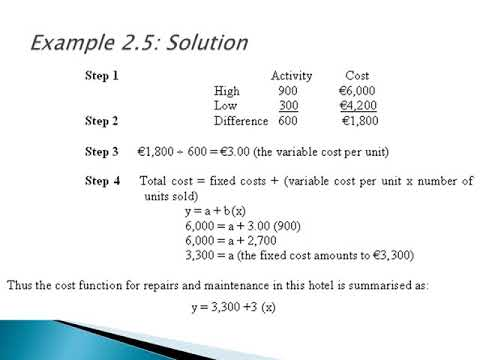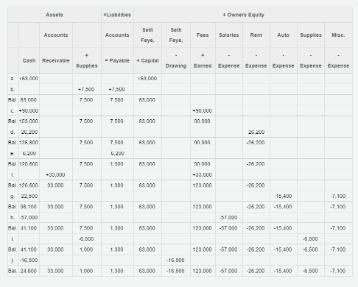# High Low Method Calculate Variable Cost Per Unit and Fixed CostThis is not only because it is simple, but also because it does not require complex tools or programs. It is important to note that if a higher level of activity is above a threshold of normal production. One has to consider step fixed cost/additional fixed cost to come up with the full fixed cost. When reviewing your company’s production and cost data, you will first have to find the highest and lowest quantity of items produced. Once you have that information, then you will be able to apply them to the formula. The high-low method is relatively unreliable because it only takes two extreme activity levels into consideration.

The high-low method separates fixed and variable costs from the total cost by analyzing the costs at the highest and lowest levels of activity. It compares the highest level of activity and the lowest level of training and then compares costs at each level. This is a very important concept in cost accounting and is very useful in determining fixed and variable costs related to the product, machinery, etc., and is also used in budgeting activities. It is a very simple method to analyze the cost without getting into complex calculations.

## What is the High-Low Method in Accounting? – Explained

Unfortunately, the only available data is the level of activity (number of guests) in a given month and the total costs incurred in each month. Being a new hire at the company, the manager assigns you the task of anticipating the costs that would be incurred in the following month (September). If the variable cost is a fixed charge per unit and fixed costs remain the same, it is possible to determine the fixed and variable costs by solving the system of equations. Variable costs will change depending on the number of units you’re producing. Unlike fixed costs, variable costs will increase when producing more units and decrease when you produce fewer. Consider the total production cost of February was USD 45,000 and the number of units produced was 10,000.Companies usually want to understand the cost structure of the products they manufacture. Hence, it is important for managers to understand what is the high-low method. In cost accounting, the high-low method is a method that attempts splitting mixed costs into fixed costs and variable costs. For mixed costs, that are also called semi-variable cost, they refer to costs that have a mixture of fixed and variable components. A business organization might be paying \$500 monthly just to keep the light and buildings operating at minimal level.

## Formulas and Examples

You will notice that the high-low method will only give you an estimate of what total costs would be at any given amount of production. These estimates are helpful to management when preparing budgets for upcoming months. In cost accounting, the high-low method is a technique used to split mixed costs into fixed and variable costs.Regression analysis helps forecast costs as well, by comparing the influence of one predictive variable upon another value or criteria. However, regression analysis is only as good as the set of data points used, and the results suffer when the data set is incomplete. This can be used to calculate the total cost of various units for the bakery. So the highest activity happened in the month of Jun, and the lowest was in the month of March.

## The Difference Between the High-Low Method and Regression Analysis

The cost amounts adjacent to these activity levels will be used in the high-low method, even though these cost amounts are not necessarily the highest and lowest costs for the year. The main advantage of the high-low method accounting formula is its simplicity. This method only requires two data points to provide estimates related to the cost structure. The high-low method in accounting is the most preferred in the case when accountants need quick information related to the cost model.

• However, for variable costs, they refer to costs that increases as the number of output increases.
• In addition to that, the high-low method allows companies to identify the cost structure, or cost model, for the goods they are producing.
• A business organization might be paying \$500 monthly just to keep the light and buildings operating at minimal level.
• When reviewing your company’s production and cost data, you will first have to find the highest and lowest quantity of items produced.

So, the differential cost of USD 10,000 divided by differential units of 4,000 results in USD 2.5 per unit (10,000/4,000). For instance, the factory got a monthly production capacity of 10,000 units and paid USD 10,000 per month. Hence, when we deduct USD 45,000 in USD 55,000, the fixed cost is net and the variable cost to the extent of equality in the level of production is eliminated. In other words, as fixed cost is the same in both months, the fixed cost has been eliminated by deduction.